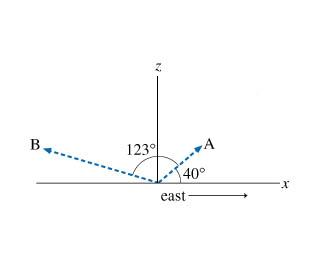# Question Solved1 AnswerA radar station, located at the origin of xz plane, as shown in the figure (Figure 1), detects an airplane coming straight at the station from the east. At first observation (point A), the position of the airplane relative to the origin is R⃗ A. The position vector R⃗ A has a magnitude of 360 m and is located at exactly 40 degrees above the horizon. The airplane is tracked for another 123 degrees in the vertical east-west plane for 5.0 s, until it has passed directly over the station and reached point B. The position of point B relative to the origin is R⃗ B (the magnitude of R⃗ B is 880 m). The contact points are shown in the diagram, where the x axis represents the ground and the positive z direction is upward. Define the displacement of the airplane while the radar was tracking it: R⃗ BA=R⃗ B−R⃗ AR→BA=R→B−R→A. What are the components of R⃗ BAR→BAR_vec_BA? 1239 40° east-A radar station, located at the origin of xz plane, as shown in the figure (Figure 1), detects an airplane coming straight at the station from the east. At first observation (point A), the position of the airplane relative to the origin is R⃗ A. The position vector R⃗ A has a magnitude of 360 m and is located at exactly 40 degrees above the horizon. The airplane is tracked for another 123 degrees in the vertical east-west plane for 5.0 s, until it has passed directly over the station and reached point B. The position of point B relative to the origin is R⃗ B (the magnitude of R⃗ B is 880 m). The contact points are shown in the diagram, where the x axis represents the ground and the positive z direction is upward.Define the displacement of the airplane while the radar was tracking it: R⃗ BA=R⃗ B−R⃗ AR→BA=R→B−R→A. What are the components of R⃗ BAR→BAR_vec_BA?

Transcribed Image Text: 1239 40° east-
More
Transcribed Image Text: 1239 40° east-